# Prediction of Shear Strength of Soil Using Direct Shear Test and Support Vector Machine Model

Hai-Bang Ly1, Binh Thai Pham1, *
1 Department of Geotechnical Engineering, University of Transport Technology, Hanoi 100000, Vietnam

#### Article Metrics

0
##### Total Statistics:

Full-Text HTML Views: 1230
Abstract HTML Views: 344
##### Unique Statistics:

Full-Text HTML Views: 582
Abstract HTML Views: 181open-access license: This is an open access article distributed under the terms of the Creative Commons Attribution 4.0 International Public License (CC-BY 4.0), a copy of which is available at: (https://creativecommons.org/licenses/by/4.0/legalcode). This license permits unrestricted use, distribution, and reproduction in any medium, provided the original author and source are credited.

* Address correspondence to this author at the Department of Geotechnical Engineering, University of Transport Technology, Hanoi, Vietnam E-mail: binhpt@utt.edu.vn

## Abstract

### Background:

Shear strength of soil, the magnitude of shear stress that a soil can maintain, is an important factor in geotechnical engineering.

### Objective:

The main objective of this study is dedicated to the development of a machine learning algorithm, namely Support Vector Machine (SVM) to predict the shear strength of soil based on 6 input variables such as clay content, moisture content, specific gravity, void ratio, liquid limit and plastic limit.

### Methods:

An important number of experimental measurements, including more than 500 samples was gathered from the Long Phu 1 power plant project’s technical reports. The accuracy of the proposed SVM was evaluated using statistical indicators such as the coefficient of correlation (R), Root Mean Squared Error (RMSE), Mean Absolute Error (MAE) over a number of 200 simulations taking into account the random sampling effect. Finally, the most accurate SVM model was used to interpret the prediction results due to Partial Dependence Plots (PDP).

### Results:

Validation results showed that SVM model performed well for prediction of soil shear strength (R = 0.9 to 0.95), and the moisture content, liquid limit and plastic limit were found as the three most affecting features to the prediction of soil shear strength.

### Conclusion:

This study might help in quick and accurate prediction of soil shear strength for practical purposes in civil engineering.

Keywords: Machine learning, Partial Dependence Plot (PDP), Root Mean Squared Error (RMSE), Support Vector Machine (SVM), Soil shear strength, Vietnam.

## 1. INTRODUCTION

Research in the field of soil mechanics has been the subject of miscellaneous studies over decades . Being considered as a discipline of civil engineering, soil mechanics is concerned with the investigation of the behavior and application of soil as materials for construction . The nature of soil mechanics involves the application of mechanical, hydraulic, or even chemical laws to deal with engineering problems. Moreover, a multiphase composition of soil containing particles, water and air, making soil unique engineering properties . Many soil-related researches have been performed, focusing on the mechanical properties , transport properties [5-7], soil consolidation [8, 9] and especially the shear behavior of soil.

Indeed, the shear strength of the soil is a very important parameter in geotechnical engineering for assessment of the stability of retaining walls, embankments and determination of the bearing capacity of highway construction foundations. The determination of this parameter is often carried out in the laboratory by different kinds of tests, such as triaxial shear test, direct shear test and unconfined compression test. However, conducting these tests usually takes time and is often costly. Thus, accurate prediction of this parameter is a crucial and important task for saving time and reducing the cost of construction projects. Numerous studies have been carried out to forecast the shear strength of soils using various approaches. Motaghedi and Eslami  introduced an analytical approach for predicting the unit cohesion (c) and friction angle (φ) in considering the bearing capacity mechanism of failure at cone tip and direct shear failure along the penetrometer sleeve. Multiple linear regression was developed by McGann et al.  to develop a Christchurch-specific empirical correlation to forecast the soil shear wave velocities (Vs) derived from the Cone Penetration Test (CPT) data. Besides, the various effects of shear strength in the disturbed zone were investigated on the time-dependent behavior . Last but not least, constitutive models were developed by Oliveira et al.  to predict the shear strength of natural soil and stabilized soil by chemical agents.

In recent decades, machine learning methods have been widely applied to solve many civil engineering [14-28], especially in geotechnical problems , [29-37]. As an example, Samui  introduced the Support Vector Regression (SVR) method to predict the friction capacity of driven piles. Kuo et al.  used Artificial Neural Network (ANN) to predict the behavior of shallow foundations, including the bearing capacity. Machine learning methods namely generalized linear (GENLIN), linear regression, classification, regression tree (CART) analysis, Chi-squared Automatic Interaction Detection (CHAID), ANN and SVR were used to identify the factors influencing the shear strength and to predict the peak friction angle of soil . Other investigations to predict the shear strength of soil were carried out by using ANN and CART techniques in the work of Kanungo et al. . Probabilistic Neural Network (PNN) for predicting different parameters of shear strength (i.e., c or φ) from different soil properties such as water content (w), plasticity index (PI), dry density (DD), gravel % (GP), sand % (SP), silt % (STP), and clay % (CP) was applied by Kiran et al. . In addition, Khan et al.  used a new model called the Functional Network (FN) to predict the residual strength of the soil. In general, there are various methods and approaches to predict the shear strength of soil. Overall, the approaches based on machine learning algorithms are superior, in terms of accuracy, compared with traditional approaches.

In this study, a popular machine learning method, namely Support Vector Machines (SVM), was proposed and applied to predict the shear strength of the soil. A database including input variables (moisture content (%), clay content (%), void ratio, plastic limit (%), liquid limit (%) and specific gravity) and output variable (shear strength of soil) of 538 samples collected from the Long Phu 1 power plant project, Soc Trang province, Vietnam was used. Popular validation indicators such as R, RMSE and MAE were used to validate the performance of the model. The dependence between the shear strength of soil and input variables was finally investigated with the help of partial dependence plots analysis.

## 2. MATERIALS AND METHODS

### 2.1. Data Collection and Preparation

In this study, experimental data from the Long Phu 1 power plant project (longitude of 9°59'07.3”N and latitude of 106°04'48.0”E), Soc Trang province, Vietnam were used. In this project, a total of 538 soil samples were collected and tested in the laboratory to determine the soil properties used for the design and construction of the project. The Union of Engineering Geology, Construction & Environment (UGCE) were the two units who carried out these laboratory tests. A total of seven variable were extracted from the project reports including one output variable (shear strength of soil) and six input variables (moisture content (%), clay content (%), void ratio, plastic limit (%), liquid limit (%) and specific gravity). Summary of the statistical values of the inputs and output are given in Table 1, whereas the correlation between input variables and output is displayed in Fig. (1). It can be seen that the moisture ratio was highly correlated with the void ratio (R = 1), followed by the plastic limit (R = 0.88), liquid limit (R = 0.78) and the shear strength of soil (R = -0.65). The void ratio correlated with the output at R = -0.64. Detailed descriptions of these variables are given in the following sections.

#### 2.1.1. Output Variable

In the simulation process, the shear strength of soil was considered as an output variable. It is a linear function of the normal stress at the time of failure  which can be expressed as below (Eq. 1):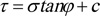(1)

where c is defined as unit cohesion (kG/cm3), φ is defined as the angle of internal friction (o), σ is defined as the normal stress on the failure plane (kG/cm3), and τ represents the shear strength of soil (kG/cm3). To calculate the shear strength of soil, the parameters such as c and φ are often determined in the laboratory through three common experiments: triaxial shear test, direct shear test, and unconfined compression test . In this study, these parameters were determined by the direct shear tests on the samples collected from the study area, and then the shear strength of soil was calculated using Eq. (1) with unit normal stress on the failure plane (σ = 1 (kG/cm3). The values of the shear strength of soil used in this study vary from 0.0368 to 0.9307 (kG/cm3) (Table 1).

#### 2.1.2. Input Variables

To predict the shear strength of soil, the input variables related to the shear strength of soil should be selected and validated. In this study, a total of six input variables considered in the prediction of the shear strength of soil included: clay content (%), moisture content (%), specific gravity, void ratio, liquid limit (%), and plastic limit (%). Description of these variables are given in the following sections:

##### 2.1.2.1. Clay Content

Particles of clay are defined as the ones with a size smaller than 0.005 mm . The content of clay affects the plasticity of the soil, and the shear strength decreases as the plasticity increases . Thus, it is reasonable to select clay content as an input variable for the prediction of shear strength in this study. Clay content (Cc), in the laboratory, is usually determined based on the analysis of grain composition by Eq. (2) :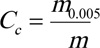(2)

where m0.005 is defined as the mass of soil particles falling through the 0.005 mm sieve size, m is defined as the mass of the soil sample. In this study, the values of clay content vary from 0.2 to 77.6% (Table 1).

##### 2.1.2.2. Moisture Content

Moisture content (Mc) is defined as the ratio in percent of the mass of water and the mass of the soil particle in the sample expressed by Eq. (3) :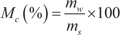(3)

where mw infers the mass of water in the soil sample and ms infers the mass of particle in the soil sample. In the laboratory, there are two common methods, namely oven drying and alcohol burning used to determine the moisture content of the soil. Moisture content affects the shear strength of soil as the higher the moisture content, the less cohesion between soil particles, and the weaker the soil becomes. In this study, the values of moisture content vary from 0.72 to 75.14% (Table 1).

##### 2.1.2.3. Specific Gravity

Specific gravity is defined as the ratio between the density of the particles and the density of the water in the soil sample  expressed by Eq. (4) :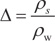(4)

where ρs infers the density of soil particles whereas ρw infers the density of water. With the soil with high specific gravity, the shear strength is also high as it contains heavy minerals, compact structure. In this study, values of specific gravity vary from 0.01 to 2.75 (Table 1).

##### 2.1.2.4. Void Ratio

Void ratio (e) - a ratio of the volume of voids to the volume of solids in the soil sample , is an important factor to evaluate the shear strength of soil as the higher the void ratio shows the lower the shear strength of soil. It can be calculated by Eq. (5) :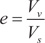(5)

where Vv infers the volume of voids in the sample whereas Vs infers the volume of the particles in the sample. In this study, the values of the void ratio vary from 0.21 to 2.089 (Table 1).

##### 2.1.2.5. Liquid Limit

Liquid Limit (LL) is known as the limited moisture at which the state of soil is changed from plastic to liquid . It affects the shear strength of soil as an increase of liquid limit leads to decreases in the shear strength of soil . Two methods, namely Cassagrande and Vasiliev, are often used to determine the liquid limit in the laboratory . It can be calculated by Eq. (6):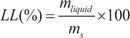(6)

where mliquid infers the mass of water in the sample at that the state of soil changed from plastic to liquid and ms infers the mass of soil particles. In this study, the values of liquid limit vary from 0.7 to 74.9% (Table 1).

##### 2.1.2.6. Plastic Limit

Plastic Limit (PL) is the limited moisture at that the state of soil is changed from solid to plastic . It affects the shear strength of soil as an increase of the plastic limit leads to a decrease in the shear strength of the soil . Atterberg tools are often used to determine the plastic limit in the laboratory , and it can be calculated by Eq. (7):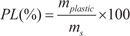(7)

where mplastic infers the mass of water in the sample at that the state of soil changed from solid to plastic and ms infers the mass of the particles in the sample. In this study, the values of plastic limit vary from 0.6 to 41% (Table 1).

## 3. SUPPORT VECTOR MACHINE

Firstly introduced by Vapnik , support vector machine (SVM) is a common machine learning method and widely used to solve many real-world problems, including soil-related properties prediction. The main concept of SVM is to map the original input space into a high-dimensional feature space by using a hyperplane [49, 50]. Let x = xi defined as a set of input factors used in the models, and y is the output (predicted variable). The SVM function is expressed by Eq. (8):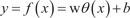(8)

where b infers the bias of the model, w is the weight matric, and θ (x) is defined as the feature mapped nonlinearly from the input space x. In this study, the choice of SVM to predict the soil shear strength was relied on many advantages of such machine learning algorithm, for instance, the ability of minimization of outliers and noise , the higher prediction capability of SVM compared with other algorithms  or the possibility to be used in a wide range of civil engineering related problems even they are highly unrelated . The SVM algorithm was coded in Matlab, based on the Machine Learning toolbox and adapted to the problem with several modifications, such as taking into account the random sampling effect or tuning the SVM parameters.

In this study, various statistical indicators, namely R, RMSE and MAE, were used to evaluate the performance of SVM. A description of these indicators can is present in the published papers [53-59]. In general, higher R illustrates the better predictive capability of the model, whereas lower RMSE and MAE show the worse predictive capability of the model [60-65].

## 4. RESULTS AND DISCUSSION

### 4.1. Prediction Performance of SVM

In the first step, the performance of SVM is evaluated in performing 200 simulations taking into the random sampling strategy to construct the training and testing datasets. As it is well-known that the data appear in the training dataset greatly affects the performance of machine learning models, the random indexing process of samples aimed to fully evaluate the performance and robustness of SVM under the presence of variability in the input space. The prediction results are evaluated by the goodness of fit between predicted and experimental values of soil shear strength. The assessment of the prediction capability is based on the goodness of fit in the testing dataset. Fig. (2) shows the statistical results for R, RMSE and MAE for testing SVM for 200 different simulations. It is worth noticing that 70% of the experimental data was randomly taken to construct the SVM model, thus the corresponding R, RMSE and MAE values were different for each simulation. As can be seen, the values of R were satisfactory and stable, ranging from R = 0.9 to 0.95, with only several outliers. Similar observations were also noticed for the values of RMSE and MAE, ranging around 0.08 (for RMSE) and 0.055 (for MAE). It can be concluded that SVM is a good predictor for estimating the shear strength of the soil.

The values of R, RMSE and MAE over 200 random sampling simulations are presented in Table 2. It was found that the SVM algorithm is a very potential predictor candidate as the variation of all error criteria was small.

The best performance of SVM, represented by the simulation, where the highest value of R was obtained with the training dataset, is presented in Fig. (3). It is observed that the predicted and experimental soil shear strength values were in good agreement, clearly proven by the satisfying relative errors (RMSE = 0.091). Only a few data points were observed as outliers, whereas the remaining results were oscillated around 0.

With respect to the testing dataset, the comparison and error are displayed in Fig. (4). As can be seen, the predicted soil shear strength values were close to the experimental ones. The relative errors were found mostly in the 10% of error with RMSE = 0.0641, close to the min values over 200 simulations. The maximum error was found as Error = -0.23, which was better than the maximum error found in the training dataset (Error = -0.58). The linear fit lines and correlation results for both training and testing parts are plotted in Fig. (5). The correlation result for the training set were R = 0.893, whereas that of the testing part was satisfying, i.e., R = 0.954.

Table 1. Summary of the statistical values of the database.
 Clay Moisture content Specific gravity Void ratio Liquid limit Plastic limit Soil shear strength Unit (mm) (%) - - (%) (%) kG/cm3 Min 0.20 0.72 0.01 0.02 0.70 0.60 0.04 Average 33.25 31.83 2.61 0.91 42.36 22.17 0.48 Median 33.20 26.55 2.69 0.79 42.50 21.40 0.50 Max 77.60 75.14 2.75 2.09 74.90 41.00 0.93 SD* 16.14 15.27 0.43 0.39 13.26 6.14 0.20
SD* = Standard deviation
Table 2. Summary of the prediction performance over 200 random simulations for the training and testing datasets.
 Part Values RMSE MAE R Error Std Train dataset Average 0.0988 0.0580 0.8824 0.0989 - Min 0.0707 0.0494 0.5088 0.0708 - Max 0.3050 0.0764 0.9399 0.3051 Test dataset Average 0.0820 0.0555 0.9164 0.0818 - Min 0.0616 0.0451 0.7220 0.0615 - Max 0.1788 0.0790 0.9537 0.1780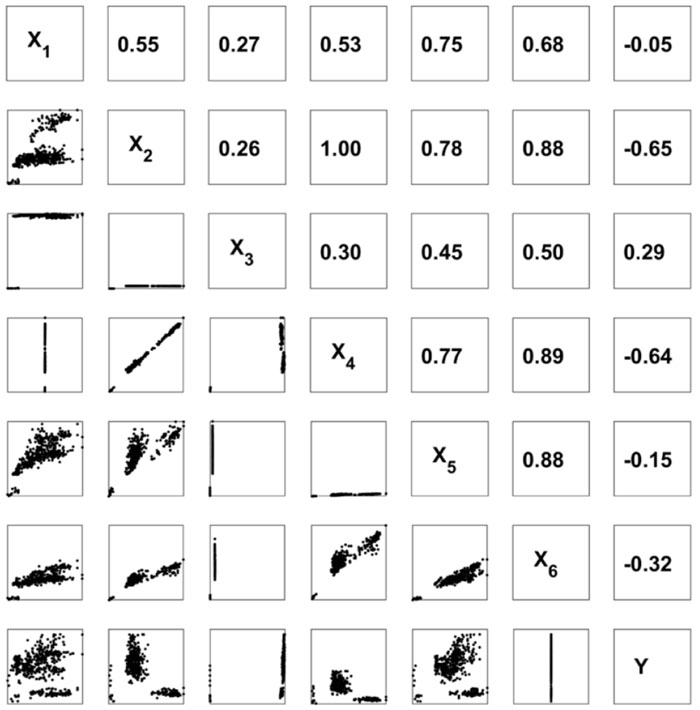Fig. (1). Correlation analysis between input variables and output in this study.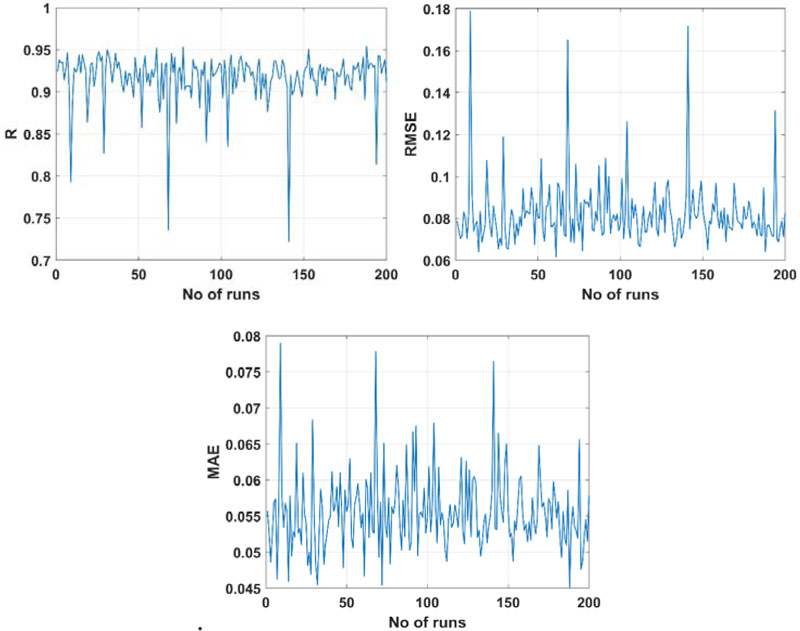Fig. (2). Results of R, RMSE, MAE for the testing part simulated by SVM for 200 simulations.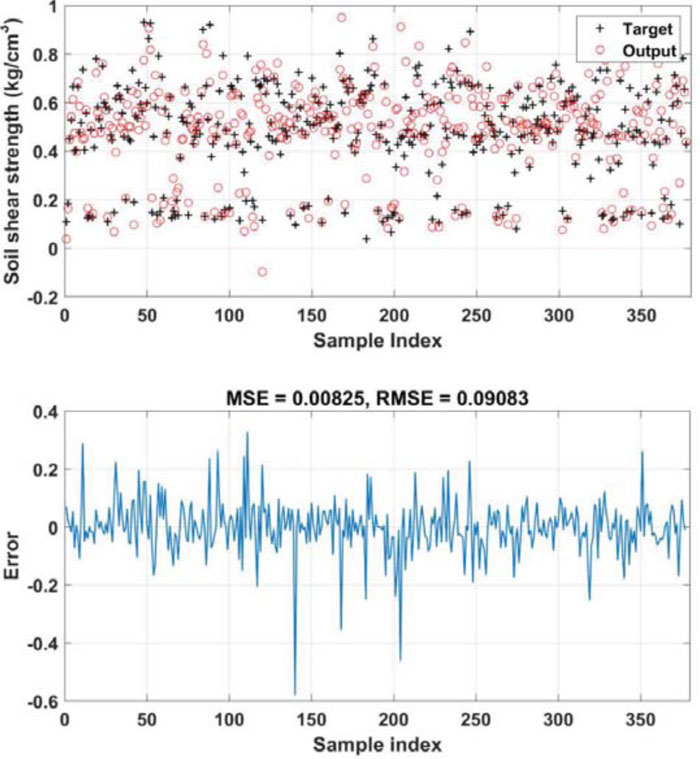Fig. (3). Comparisons between predicted and experimental soil shear strength for the training dataset and the corresponding relative errors.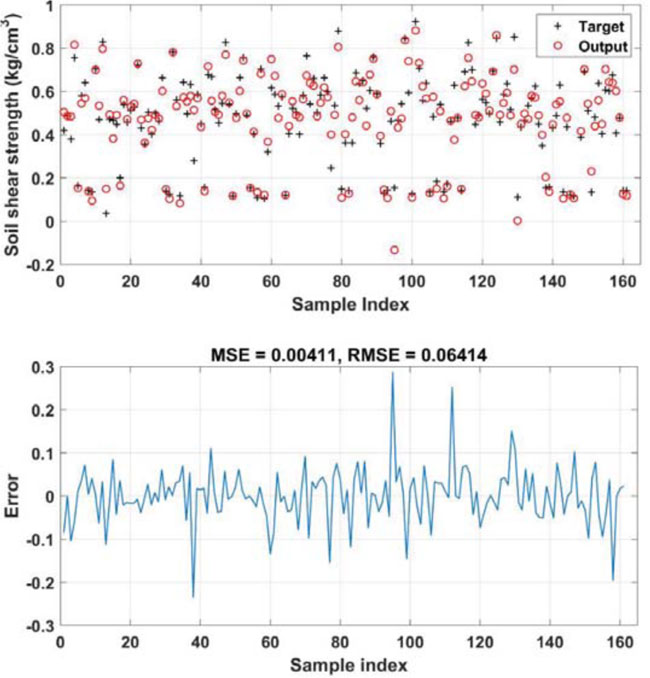Fig. (4). Comparisons between predicted and experimental soil shear strength for the testing dataset and the corresponding relative errors.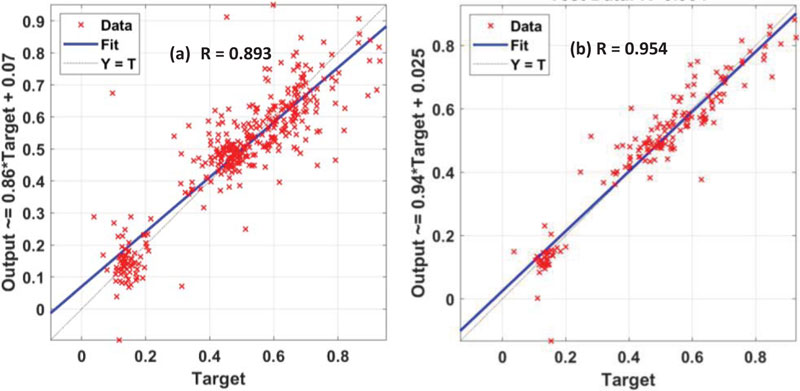Fig. (5). Correlation analysis between input variables and output in this study: (a) Training dataset and (b) testing dataset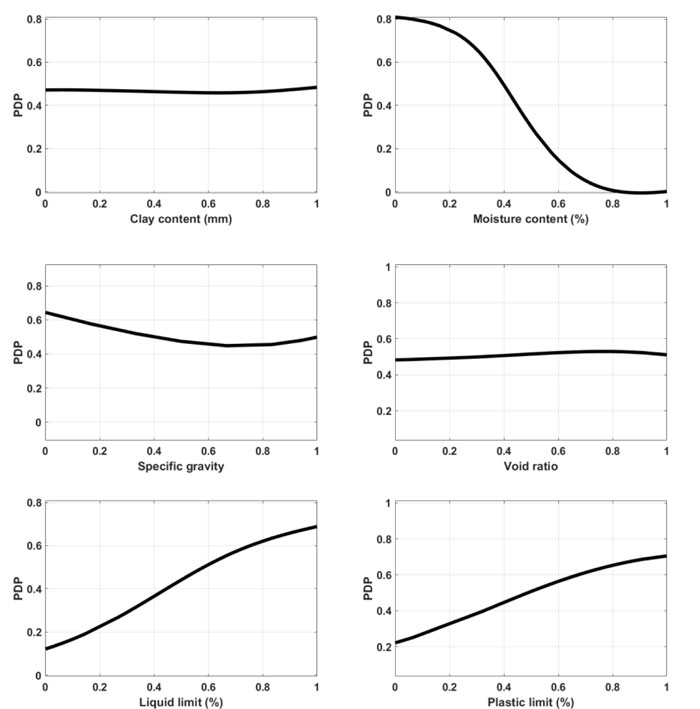Fig. (6). Partial dependence plots (PDP) of the input variables used in this study.

In the discussion, Dao et al.  predicted the mechanical properties of geopolymer concrete using ANN. Although the method gave satisfactory values of R2 around 0.75, it was also noticed in many cases that these values were close to 0. In another attempt, Nguyen et al.  compared the performance of SVM with two hybrid machine learning methods to simulate the Marshall properties of stone matrix asphalt mixtures. The SVM performance over 1000 simulations was found superior to the other hybrid artificial intelligence methods, especially no negative values of R were found. Thus, the use of SVM in this study was reasonable and the results were proven to be stable and reliable.

### 4.2. Importance of Input Factors Using Partial Dependence Plots (PDP)

Partial dependence plots (PDP) is an efficient way to represent the dependence between the target response of machine learning algorithm and a set of the selected input variable, marginalizing over the values of remaining variables to evaluate the importance of input factors to be selected for better prediction problems. In this study, PDP of the 6 input variables was derived from the best configuration of SVM, as presented in Fig. (6).

The clay content and the void ratio were found to be the less important variables throughout PDP analysis, as the variation of the predicted soil shear strength was insignificant. The value of the latter was varied from 0.4585 to 0.4839 with respect to clay content and from 0.4827 to 0.5295 with respect to the void ratio. Considering now the specific gravity, the predicted output ranged in the 0.4486 to 0.6409 range, classified as a more important variable comparing with the clay content and void ratio. The liquid limit was considered to have more effect than the plastic limit to the predicted shear strength of soil, as the latter varied from 0.2218 to 0.7051 (for plastic limit) and from 0.1216 to 0.6885 (for liquid limit). Finally, when varying the moisture content, the value of soil shear strength was found significantly fluctuated, from 0.035 to 1. It could be concluded that the order of importance of the variables in this study could be: moisture content > liquid limit > plastic limit > specific gravity > void ratio > clay content.

In general, the factors related to water were found as the important variables for the prediction of soil shear strength. The presence of water in soil reduces the friction and link among particles, thus reducing the shear strength of soil. The PDP results and classification were found in good agreement with previously published works in the literature [67, 68].

## CONCLUSION

Prediction and analysis of soil shear strength, an important parameter in geotechnical engineering, has been investigated in this study. To this aim, the development of a machine learning algorithm, SVM was conducted. The database used to predict the shear strength of soil contained 6 input variables, namely the clay content, moisture content, specific gravity, void ratio, liquid limit and plastic limit. The accuracy of the proposed SVM was successfully proved via statistical error criteria such as R, RMSE and MAE over 200 simulations. Finally, the most accurate SVM model was selected for the interpretation of the results using partial dependence plots (PDP). Validation results showed that SVM models performed well for prediction of soil shear strength (R = 0.9 to 0.95), and the moisture content, liquid limit and plastic limit were found as the three most affecting features to the prediction of soil shear strength.

In machine learning problems, data is crucial to construct a reliable prediction tool. Gathering an additional dataset is one perspective of this study. Moreover, the accuracy improvement of the prediction algorithm is also critical and will be shortly investigated, which helps avoid costly on-field experiments.

Not applicable.

None.

### FUNDING

This research is funded by Ministry of Transport, project title "Building Big Data and development of machine learning models integrated with optimization techniques for prediction of soil shear strength parameters for construction of transportation projects" under grant number DT 203029.

### CONFLICT OF INTEREST

The authors declare no conflict of interest, financial or otherwise.

## ACKNOWLEDGEMENTS

We thank Dr Manh Duc Nguyen, University of Transport Technology for providing us the data used in this study.